# MA.8.NSO.1.7Export Print
Solve multi-step mathematical and real-world problems involving the order of operations with rational numbers including exponents and radicals.

### Examples

The expression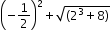is equivalent to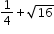which is equivalent to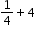which is equivalent to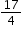.

### Clarifications

Clarification 1: Multi-step expressions are limited to 6 or fewer steps.

Clarification 2: Within this benchmark, the expectation is to simplify radicals by factoring square roots of perfect squares up to 225 and cube roots of perfect cubes from -125 to 125.

General Information
Subject Area: Mathematics (B.E.S.T.)
Strand: Number Sense and Operations
Status: State Board Approved

## Benchmark Instructional Guide

### Terms from the K-12 Glossary

• Exponents
• Rational Numbers

### Vertical Alignment

Previous Benchmarks

Next Benchmarks

### Purpose and Instructional Strategies

In grade 7, students solved mathematical problems using multi-step order of operations with rational numbers including whole-number exponents and absolute value. In grade 8, students continue to solve multi-step problems involving the order of operations with rational numbers but including integer exponents and radicals. In Algebra 1, students will solve problems with numerical radicals.
• Instruction includes providing a structure for students to track steps as they work through problems (MTR.5.1). Students should show the equivalence from one step to another to further their understanding.
• Avoid mnemonics, such as PEMDAS, that do not account for other grouping symbols and do not exercise proper number sense that allows for calculating accurately in a different order.
• Instruction includes the use of technology to help emphasize the proper use of grouping symbols for order of operations.
• Students should have experience using technology with radicals, decimals and fractions as they occur in the real world. This experience will help to students working with irrational numbers in this grade level.

### Common Misconceptions or Errors

• Students may confuse square roots with cube roots.
• Some students may incorrectly apply the order of operations. To address this misconception, be sure to review operations with rational numbers and order of operations.
• Students may incorrectly perform operations with the numbers in the problem based on what has recently been taught, rather than what is most appropriate for a solution. To address this misconception, have students estimate or predict solutions prior to solving and then compare those predictions to their actual solution to see if it is reasonable (MTR.6.1).
• Students may incorrectly oversimplify a problem by circling the numbers, underlining the question, boxing in key words, and eliminating important contextual information that may seem unimportant. This process can cause students to not be able to comprehend the context or the situation (MTR.2.1, MTR.4.1, MTR.5.1, MTR.7.1). Teachers and students should engage in questions such as:
• What do you know from the problem?
• What is the problem asking you to find?
• Are you putting groups together? Taking groups apart? Or both?
• Are the groups you are working with the same sizes or different sizes?
• Can you create a visual model to help you understand or see patterns in your problem?”

### Strategies to Support Tiered Instruction

• Teacher provides opportunities for students to comprehend the context or situation by engaging in questions (laminating these questions on a printed card for students to utilize as a resource in and out of the classroom would be helpful).
• What do you know from the problem?
• What is the problem asking you to find?
• Can you create a visual model to help you understand or see patterns in your problem?
• Instruction includes the use of colors to highlight each step of the process used to evaluate an expression.
• For example, when evaluating (−$\frac{\text{1}}{\text{3}}$)² − $\sqrt{2² + 4}$ students can first highlight the grouping with any exponents, roots or parenthesis: (−$\frac{\text{1}}{\text{3}}$$\sqrt{2² + 4}$. Then, students can determine any order of operations within each of those larger groupings. Students should see that within the cube root, they can perform 2² + 4 and that they can perform (−$\frac{\text{1}}{\text{3}}$. Students could have the expression $\frac{\text{1}}{\text{9}}$$\sqrt{8}$, and then perform $\sqrt{8}$ to obtain $\frac{\text{1}}{\text{9}}$ − 2 which is equivalent to −$\frac{\text{17}}{\text{9}}$.
• Instruction includes the use a three-read strategy. Students read the problem three different times, each with a different purpose (laminating these questions on a printed card for students to utilize as a resource in and out of the classroom would be helpful).
• First, read the problem with the purpose of answering the question: What is the problem, context, or story about?
• Second, read the problem with the purpose of answering the question: What are we trying to find out?
• Third, read the problem with the purpose of answering the question: What information is important in the problem?
• Teacher has students estimate or predict solutions prior to solving and then compare those predictions to their actual solution to see if it is reasonable (MTR.6.1).

The Dotson’s family was designing their backyard to be a peaceful sanctuary with areas
dedicated to working out, a swimming pool and a gazebo. Each space is a square design having the same size. The total backyard area is 600 square feet. The Dotson’s want to fence the outside of their property but will not fence what is up against the house. The diagram below shows the layout of the backyard.
• Part A. How much fencing, in feet, would the Dotson’s need to purchase to fence in the property?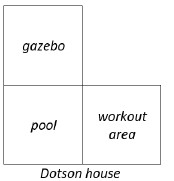• Part B. The Dotson’s went to Fence2Fence and found the following options for purchase:
• 3$\frac{\text{1}}{\text{2}}$ feet ×6 feet Western Red Cedar Gothic Fence Panels for \$60.05
• 3$\frac{\text{1}}{\text{2}}$ feet ×8 feet Western Red Cedar Essentials Fence Panels for \$88.66
Which option is the better value? Why?

### Instructional Items

Instructional Item 1
Calculate the value of the expression given.
$\sqrt{27}$ − 1.4 ($\sqrt{3² - 5}$)

*The strategies, tasks and items included in the B1G-M are examples and should not be considered comprehensive.

## Related Courses

This benchmark is part of these courses.
1200400: Foundational Skills in Mathematics 9-12 (Specifically in versions: 2014 - 2015, 2015 - 2022, 2022 and beyond (current))
1205050: M/J Accelerated Mathematics Grade 7 (Specifically in versions: 2014 - 2015, 2015 - 2020, 2020 - 2022, 2022 and beyond (current))
1205070: M/J Grade 8 Pre-Algebra (Specifically in versions: 2014 - 2015, 2015 - 2022, 2022 and beyond (current))
1204000: M/J Foundational Skills in Mathematics 6-8 (Specifically in versions: 2014 - 2015, 2015 - 2022, 2022 and beyond (current))
7812030: Access M/J Grade 8 Pre-Algebra (Specifically in versions: 2014 - 2015, 2015 - 2018, 2018 - 2019, 2019 - 2022, 2022 and beyond (current))

## Related Access Points

Alternate version of this benchmark for students with significant cognitive disabilities.
MA.8.NSO.1.AP.7: Use tools to solve multi-step mathematical problems, with four or fewer steps, involving the order of operations with rational numbers including exponents and perfect squares and/or square roots.

## Related Resources

Vetted resources educators can use to teach the concepts and skills in this benchmark.

## Formative Assessment

Dimensions Needed:

Students are asked to solve problems involving square roots and cube roots.

Type: Formative Assessment

## Original Student Tutorial

Square Root Part 3: Simplifying Radicals:

Learn how to simplify radicals in this interactive tutorial.

Type: Original Student Tutorial

## MFAS Formative Assessments

Dimensions Needed:

Students are asked to solve problems involving square roots and cube roots.

## Original Student Tutorials Mathematics - Grades 6-8

Square Root Part 3: Simplifying Radicals:

Learn how to simplify radicals in this interactive tutorial.

## Student Resources

Vetted resources students can use to learn the concepts and skills in this benchmark.

## Original Student Tutorial

Square Root Part 3: Simplifying Radicals:

Learn how to simplify radicals in this interactive tutorial.

Type: Original Student Tutorial

## Parent Resources

Vetted resources caregivers can use to help students learn the concepts and skills in this benchmark.